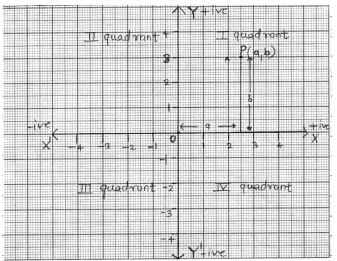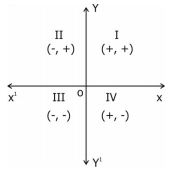1. /
2. CBSE
3. /
4. Class 09
5. /
6. Coordinate Geometry class 9...

# Coordinate Geometry class 9 Notes Mathematics### myCBSEguide App

Download the app to get CBSE Sample Papers 2023-24, NCERT Solutions (Revised), Most Important Questions, Previous Year Question Bank, Mock Tests, and Detailed Notes.

CBSE class 9 Mathematics Chapter 3 Coordinate Geometry notes in PDF are available for free download in myCBSEguide mobile app. The best app for CBSE students now provides Coordinate Geometry class 9 Notes latest chapter wise notes for quick preparation of CBSE exams and school based annual examinations. Class 9 Mathematics notes on Chapter 3 Coordinate Geometry are also available for download in CBSE Guide website.

## CBSE Guide Coordinate Geometry class 9 Notes

CBSE guide notes are the comprehensive notes which covers the latest syllabus of CBSE and NCERT. It includes all the topics given in NCERT class 9 Mathematics text book. Users can download CBSE guide quick revision notes from myCBSEguide mobile app and my CBSE guide website.

## 9 Mathematics notes Chapter 3 Coordinate Geometry

Download CBSE class 9th revision notes for Chapter 3 Coordinate Geometry in PDF format for free. Download revision notes for Coordinate Geometry class 9 Notes and score high in exams. These are the Coordinate Geometry class 9 Notes prepared by team of expert teachers. The revision notes help you revise the whole chapter in minutes. Revising notes in exam days is on of the best tips recommended by teachers during exam days.

CBSE Class 09  Mathematics
Revision Notes
CHAPTER – 3
COORDINATE GEOMETRY

1. Cartesian System
2. Plotting a Point in the Plane with given Coordinates

Coordinate Geometry : The branch of mathematics in which geometric problems are solved through algebra by using the coordinate system is known as coordinate geometry.

Coordinate System

Coordinate axes: The position of a point in a plane is determined with reference to two fixed mutually perpendicular lines, called the coordinate axes.In this system, position of a point is described by ordered pair of two numbers.

Quadrants: The coordinate axes divide the plane into four parts which are known as quadrants.

Ordered pair : A pair of numbers a and b listed in a specific order with ‘a’ at the first place and ‘b’ at the second place is called an ordered pair (a,b)

Note that
Thus (2,3) is one ordered pair and (3,2) is another ordered pair.
In given figure O is called origin.
The horizontal line
XOX’ is called the -axis.
The vertical line YOY’ is called the -axis.
P(a,b) be any point in the plane. ‘a’ the first number denotes the distance of point from
-axis and ‘b’ the second number denotes the distance of point from -axis.
a – X – coordinate | abscissa of P.
b – Y – coordinate | ordinate of P.
The point of intersection of the coordinate axes is called the origin.
The coordinates of origin are (0,0)
Every point on the x-axis is at a distance o unit from the -axis. So its ordinate is 0.
Every point on the y-axis is at a distance of unit from the -axis. So, its abscissa is 0.Note : Any point lying on – axis or – axis does not lie in any quadrant.
The sign of coordinates (x, y) of a point in various quadrant are as given below:

 Quadrant Coordinates x y I + + II – + III – – IV + –

## Coordinate Geometry class 9 Notes

• CBSE Revision notes for Class 9 Mathematics PDF
• CBSE Revision notes Class 9 Mathematics – CBSE
• CBSE Revisions notes and Key Points Class 9 Mathematics
• Summary of the NCERT books all chapters in Mathematics class 9
• Short notes for CBSE class 9th Mathematics
• Key notes and chapter summary of Mathematics class 9
• Quick revision notes for CBSE exams

## CBSE Class-9 Revision Notes and Key Points

Coordinate Geometry class 9 Notes. CBSE quick revision note for Class-9 Mathematics, Chemistry, Maths, Biology and other subject are very helpful to revise the whole syllabus during exam days. The revision notes covers all important formulas and concepts given in the chapter. Even if you wish to have an overview of a chapter, quick revision notes are here to do if for you. These notes will certainly save your time during stressful exam days.

To download Coordinate Geometry class 9 Notes, sample paper for class 9 Mathematics, Social Science, Science, English Communicative; do check myCBSEguide app or website. myCBSEguide provides sample papers with solution, test papers for chapter-wise practice, NCERT solutions, NCERT Exemplar solutions, quick revision notes for ready reference, CBSE guess papers and CBSE important question papers. Sample Paper all are made available through the best app for CBSE students and myCBSEguide website.### Test Generator

Create question paper PDF and online tests with your own name & logo in minutes.### myCBSEguide

Question Bank, Mock Tests, Exam Papers, NCERT Solutions, Sample Papers, Notes